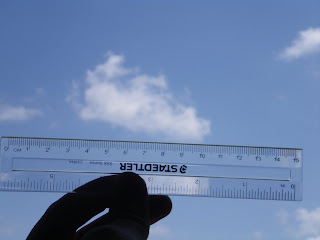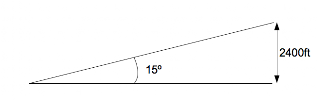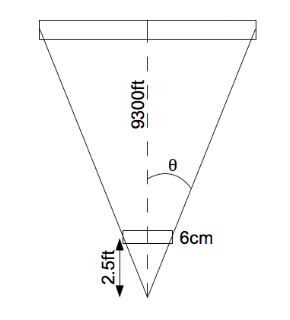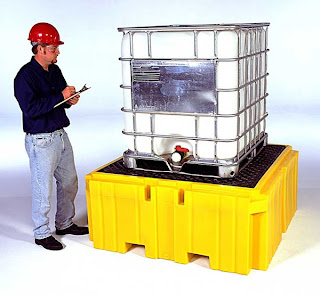### How much does a cloud weigh?

I was chatting with a friend not long ago, and he mentioned that he sometimes pictured clouds as these malevolant, multi-ton monstrosities hovering overhead, just waiting to smash down on us tiny humans. And by the way, how much does a cloud actually weigh?

Clearly, this calls for some math: I decided to calculate how much a cloud actually massed.

I started out by finding a cloud that I could measure reasonably well.Obviously, this photo by itself tells me nothing about the size of the cloud. However, I combined the 6cm apparent size of the cloud with an angle measurement, using my oh-so-sophisticated home-made astrolabe and the reported cloud heights from a nearby airport. (I made sure to choose a cloud that was over an airport: their weather reports are very accurate, include cloud heights to the nearest 100ft, and are very local.)The astrolabe I used is not the most accurate instrument, so the following calculations are pretty approximate.

The first step was to calculate the distance to the cloud. Using the measured angle and the known cloud height, trigonometry gives the answer very easily.The distance to cloud is the hypotenuse, which I can calculate using:

$\sin 15^\circ = {2400\mathrm{ft}\over x}$ $x = {2400\mathrm{ft}\over \sin15^\circ} \approx 9300\mathrm{ft}$

Now that we know the distance to the cloud, the 6cm on the ruler can be related to the actual size of the cloud. Again, trigonometry works its magic:We don't know the angle, but that's ok, because I know the distance between my camera and my ruler, roughly 2.5ft, the distance between my camera and the cloud, roughly 9300ft, and the measurement on the ruler, 6cm. To make a right triangle and make the tangent work, I'm splitting the cloud and the ruler in half, as indicated by the dashed line. So I'm calculating half the width of the cloud based on half of the 6cm measurement.

The angle $$\theta$$ is therefore given by:

$\tan\theta = {3\mathrm{cm}\over 2.5\mathrm{ft}}$

We won't bother calculating $$\theta$$, because the half-width of the cloud uses the same angle, given by:

$\tan\theta = {x\over 9300\mathrm{ft}}$

Since the angle is the same we can combine these two equations to eliminate it:

${3\mathrm{cm}\over 2.5\mathrm{ft}} = {x\over 9300\mathrm{ft}}$

And yes, I can mix and match units like that. The adjacent measurement in both is in feet, and the opposite measurement in both will be in cm. The units of ft cancel out leaving only cm; this is all about ratios anyway.

The half-width of the cloud is therefore 11160cm, or 111.6m. Or, given the accuracy of the preceding calculations, approximately 110m. The full width of the cloud would then be approximately 220m.

The height of the cloud can be calculated using the same equation, using an estimate of 3.5cm height at the high side and 1.5cm height at the low side to give 13,020cm and 5580cm. A little bit of geometry later (220m x 56m rectangle plus 220m x 74m triangle) and we get a visible area of 20,460m2.

Since I have absolutely no idea about the depth of the cloud, I will assume for the purposes of this calculation that the base is square and I'm looking straight at one side: that is, the cloud is about 220m side to side as I see it, so is also 220m front to back. This gives us a total volume of cloud of approximately 4,500,000m3. For comparison, the white container inside the steel cage is about 1m3.Now that we know the approximate volume of the cloud, we need the liquid density inside the cloud, to figure out how much it masses.

Obviously a cloud isn't entirely water, or it really would crash down on us and destroy a lot of things when it landed; water weighs 1000 kg for every cubic metre, and that cloud is several million cubic metres in size. They're made up of lots of tiny droplets of water, so to find out how much water is in the cloud, I have to find out both how many droplets per m3 there are, and how big those droplets are.

I found a cloud simulation paper that had some characteristic values for different types of clouds, and used those. Specifically, it described continental, clean cumulus clouds (as opposed to maritime, or polluted, or stratus or cirrus) as having a characteristic particle radius of 5.77$$\mu$$m and a typical particle density of 400 particles/cm3.

Using these numbers, the volume of a single characteristic droplet is:

$V = {4\over3}\pi r^3 = {4\over3}\pi 5.77\times10^{-4}\mathrm{cm}^3 = 8.05\times10^{-10}\mathrm{cm}^3$

As there are 400 particles/cm3, this gives us 3.2x10-7 cm3 water per cm3 of cloud. This is much less then 1%. In fact, it's less than 1 part per million by volume. This explains why airplanes can fly through clouds and the only problem they have is that the pilots can't see anything; it's like driving in thick fog.

But how much water is in the entire cloud?

$4,500,000m^3 \times (3.2\times10^{-7}\mathrm{g}/\mathrm{cm}^3 \times {(100\mathrm{cm})^3 \over \mathrm{m}^3} \approx 1440\mathrm{kg}$

Almost a ton and a half of water in that little cloud! Maybe my friend was right.

But as big as that sounds, we can't forget that air has mass too, even if it is much lower density than water. At the altitude of that cloud, about 2400ft, the density of air is 1.1kg/m3.

The mass of air in the volume occupied by that cloud is therefore:

$4,500,000\mathrm{m}^3 \times 1.1\mathrm{kg}/\mathrm{m}^3 \approx 4,950,000\mathrm{kg}$

Or, 5000 tonnes. The water is about 0.03% of the total mass of the cloud, and whether the cloud is there or not, the air will be.